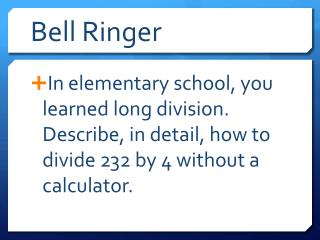Download PresentationBell Ringer

# Bell Ringer - PowerPoint PPT PresentationDownload Presentation## Bell Ringer

- - - - - - - - - - - - - - - - - - - - - - - - - - - E N D - - - - - - - - - - - - - - - - - - - - - - - - - - -
##### Presentation Transcript

1. Bell Ringer • In elementary school, you learned long division. Describe, in detail, how to divide 232 by 4 without a calculator.

2. Dividing Polynomials Tuesday, September 16, 2014

3. Long Division For Polynomials

4. The 1st way may be what you are used to seeing. The 2nd way is written like a rational function. Just like fractions, we can split it up. Then we can follow the rules of exponents. • 6xy2 - 3xy + 2x2y xyxyxy • 6y -3 + 2x Divide a Polynomial by a Monomial Ex: (6xy2 – 3xy + 2x2y) ÷xy Is the same as: 6xy2– 3xy + 2x2y xy

5. You Try • (5ab2 – 4ab + 7a2b) (ab)-1 • Hint – First make the negative exponent positive.

6. Just like in elementary school… • Divide • Multiply • Subtract • Bring Down • If there is a remainder… write it as a fraction. • Like with decimals, you may need a placeholder. Divide a Polynomial by a Binomial Ex: (x2 – 10x – 24 ) ÷ (x + 2) (8x4– 4x2 + x +4) ÷ (2x + 1) (x3 + y3) ÷ (x + y)

7. You Try • 1. (3a4 – 6a3 – 2a2 + a – 6) ÷ (a + 1) • 2. (t2 + 3t – 9) ÷ (5 – t ) Hint: reorder the denominator • 3. (3x3 – 5x2 + 10x – 3) ÷ (3x + 1)

8. Synthetic Division For Polynomials

9. You can only use synthetic division when dividing by a binomial that is of degree 1 with a coefficient of 1. • IF the coefficient is not 1, you can divide the polynomial and binomial by the coefficient and then use synthetic division, but it’s messy, so you might as well use long division. Divide a Polynomial by a Binomial Ex: 1. (5x3 – 13x2 + 10x – 8) ÷ (x – 2) 2. (x3 + 13x2 – 12x – 8) ÷ (x + 2) Write the coefficients. Put the opposite of the constant of the divisor in the box. Bring down, multiply, add, repeat. Write your answer (1 degree less than the dividend).

10. You Try • 1. (b4 – 2b3 + b2 – 3b + 2) ÷ (b – 2) • 2. (x2 – 4x + 6) ÷ (x – 3)

11. ClassworkAlgebra II book p. 236-237 #16-54 even

12. HomeworkPolynomial Division Worksheet

13. Exit Ticket1. By a show of hands, who prefers synthetic division?2. Why?